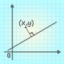Hlavní navigace

#MathTool Line Point Graph 1.3

This is an advertisement supported math tool. It accepts two different simple geometry objects - either line or point as input. There are six ways to input a line. You will get different additional information (no detail steps provided) depend on the combination of input. You can also plot the inputs on a simple graph.

Number input/display format:
* Decimal
* Fraction (distance will be displayed in decimal)
(enter 2/3 by pressing [a b/c], 1 2/3 by pressing [a b/c][a b/c], where key [a b/c] will show up in fraction display mode)

Line input format:
* General From --> ax + by + c = 0
* Slope Intercept Form --> y = mx + c
* Point Slope Form --> (y - y1) = m(x - x1)
* Line By Two Points --> (x1, y1) - (x2, y2)
* Horizontal Line --> y = c
* Vertical Line --> x = c

Point & Point:
- mid-point
- distance
- line from two points
- slop of line
- x-intercept of line
- y-intercept of line

Point & Line:
- distance from point to line
- parallel line through point
- perpendicular line from point to line
- intercepting point of perpendicular line to given line

Line & line:
- check if they are parallel
- check if they are perpendicular
- intercepting point of two lines

## Celkové hodnocení

Průměr hodnocení
3

Pro hodnocení programu se prosím nejprve

## Souhrnné informace o MathTool Line Point Graph

1.3

ano

6,3 MB

• Angličtina
• #### Staženo

0× celkem
0× tento měsíc

9. 10. 2019

## Něco jsme propásli?

Dejte nám vědět. Upozornit redakci Stahuj
Velice děkujeme za Vaše podněty